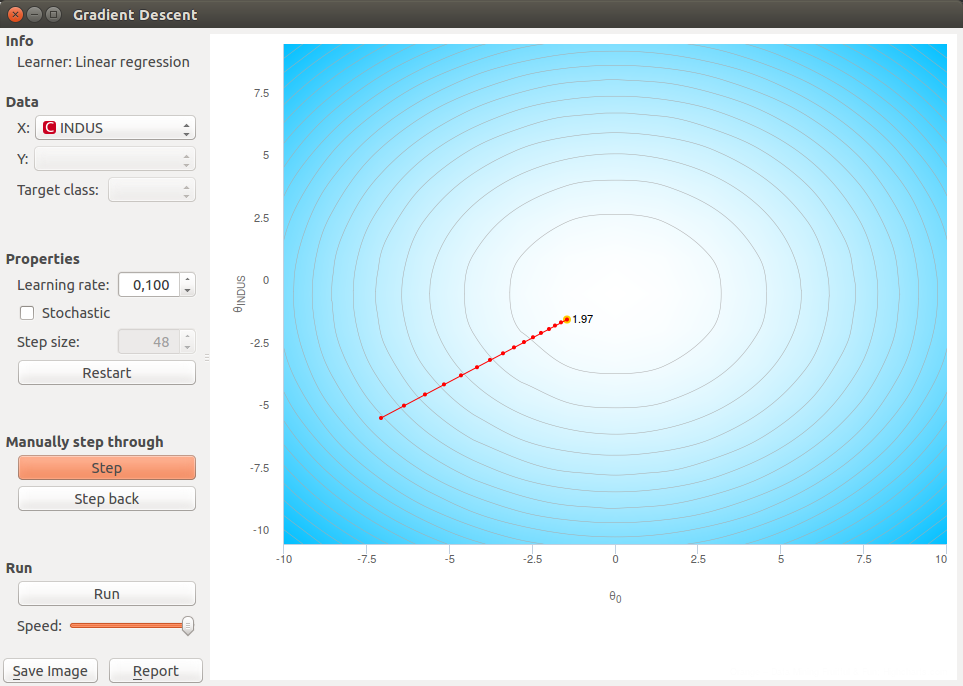Educational widget that shows gradient descent algorithm on a logistic or linear regression.

Signals¶

Inputs:

• Data

Input data set.

Outputs:

• Data

Data with columns selected in widget.

• Classifier

Model produced on the current step of the algorithm.

• Coefficients

Logistic regression coefficients on the current step of the algorithm.

Description¶

This widget shows steps of gradient descent for a logistic and linear regression step by step. Gradient descent is demonstrated on two attributes that are selected by user.

Gradient descent is performed on logistic regression if class in data set is discrete and linear regression if class is continuous.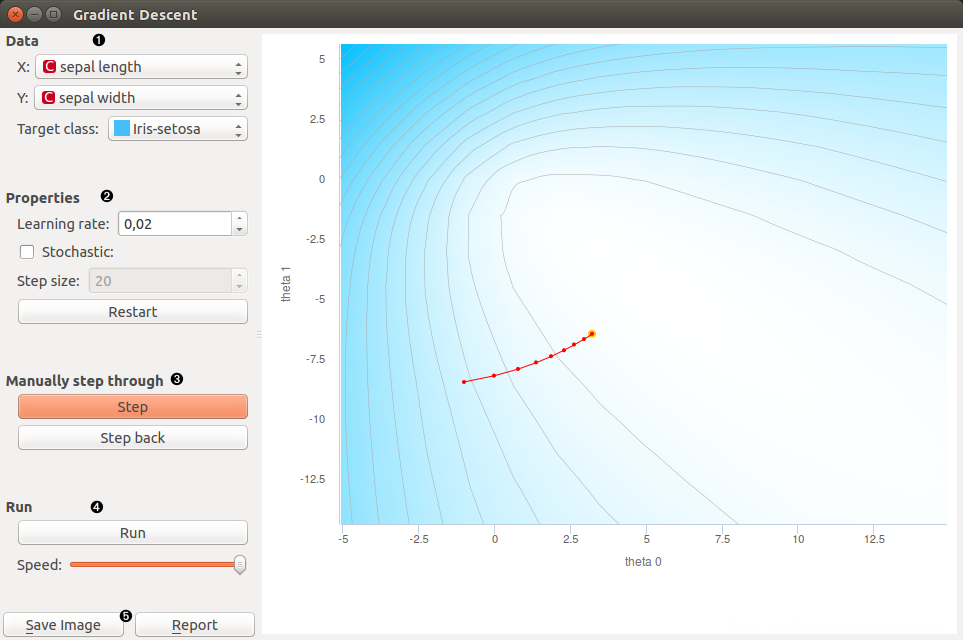1. Select two attributes (x and y) on which gradient descent algorithm is preformed. Select target class. It is class that is classified against all other classes.

2. Learning rate is step size in a gradient descent

With stochastic checkbox you can select whether gradient descent is stochastic or not. If stochastic is checked you can set step size that is amount of steps of stochastic gradient descent performed in one press on step button.

Restart: start algorithm from beginning

3. Step: perform one step of the algorithm

Step back: make a step back in the algorithm

4. Run: automatically perform several steps until algorithm converge

Speed: set speed of automatic stepping

5. Save Image saves the image to the computer in a .svg or .png format.

Report includes widget parameters and visualization in the report.

Example¶

In Orange we connected File widget with Iris data set to Gradient Descent widget. Iris data set has discrete class so Logistic regression will be used this time. We connected outputs of the widget to Predictions widget to see how data are classified and Data Table widget where we inspect coefficients of logistic regression.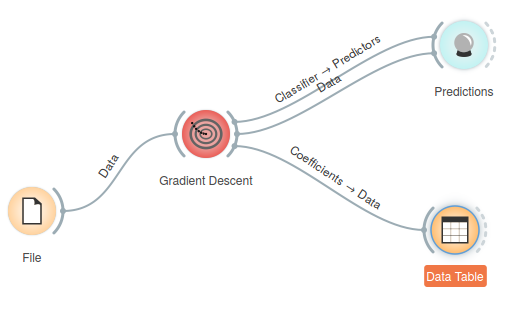We opened Gradient Descent widget and set X to sepal width and Y to sepal length. Target class is set to Iris-virginica. We set learning rate to 0.02. With click in graph we set beginning coefficients (red dot).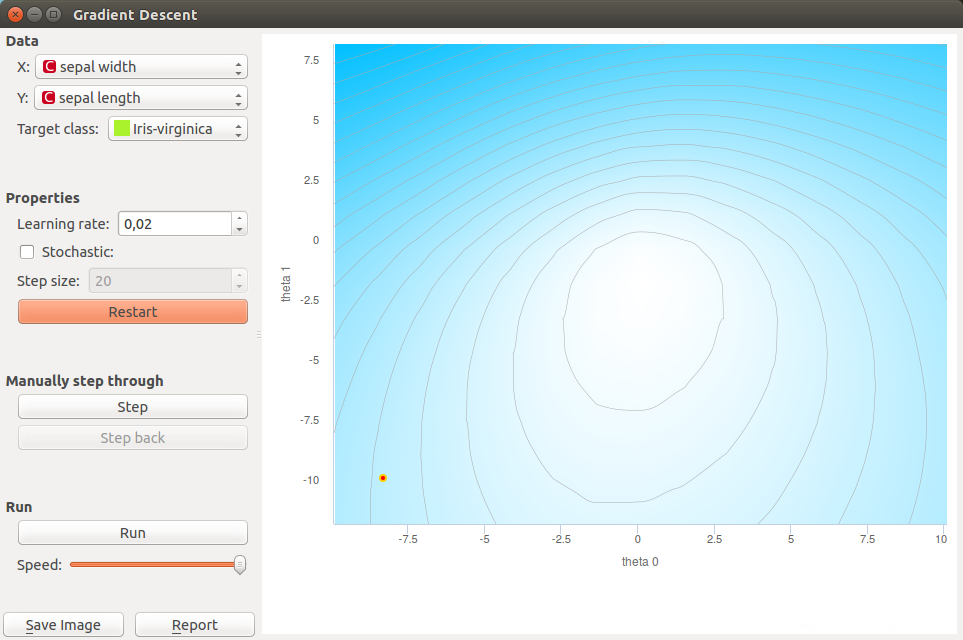We performs step of the algorithm with pressing Step button. When we get bored with clicking we can finish stepping with press on Run button.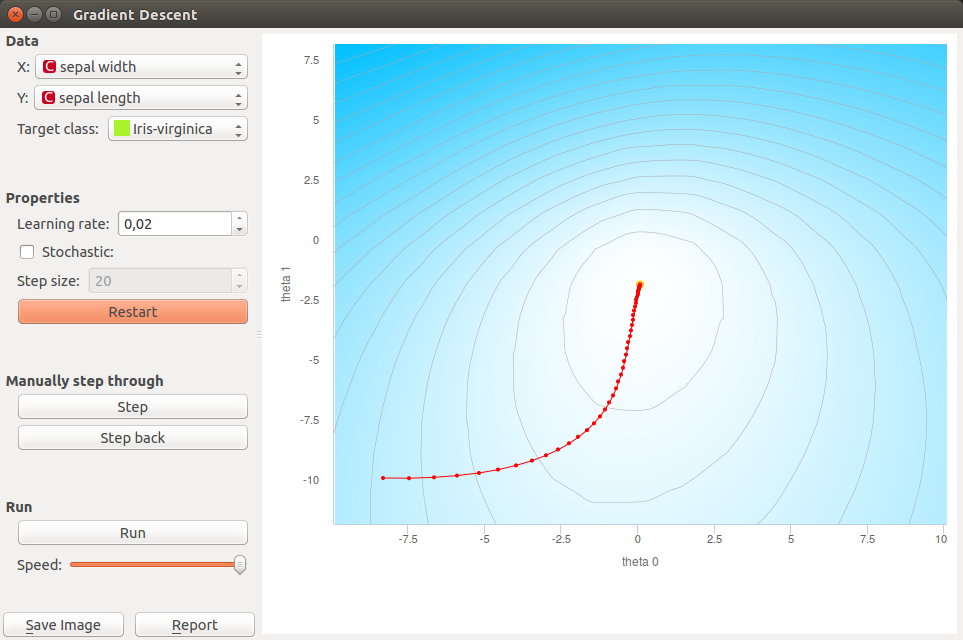If we want to go back in the algorithm we can do it with pressing Step back button. This will also change model. Current model uses positions of last coefficients (red-yellow dot).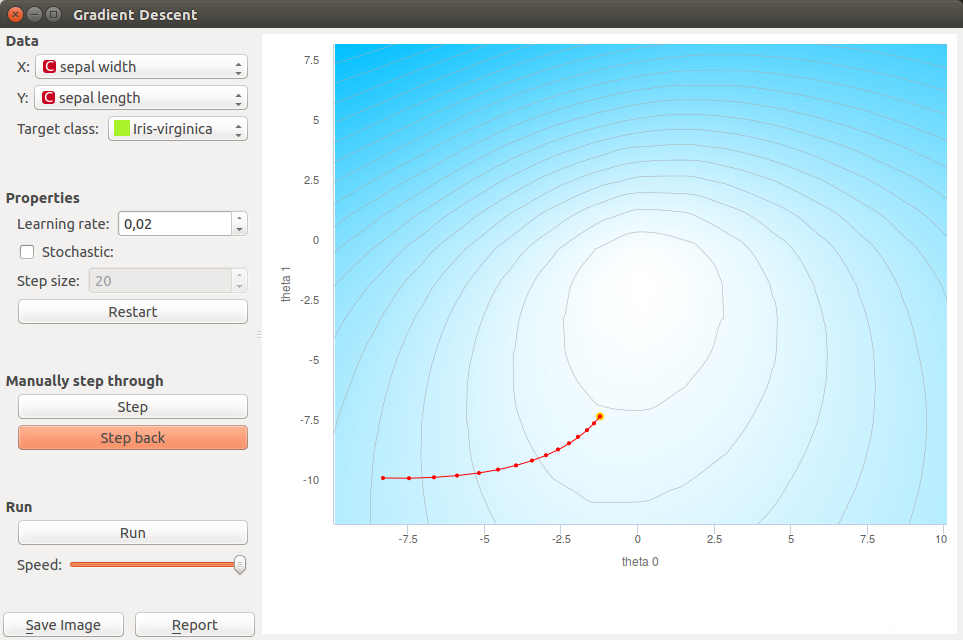In the end we want to see predictions for input data so we can open Predictions widget. Predictions are listed in left column. We can compare this predictions to real classes.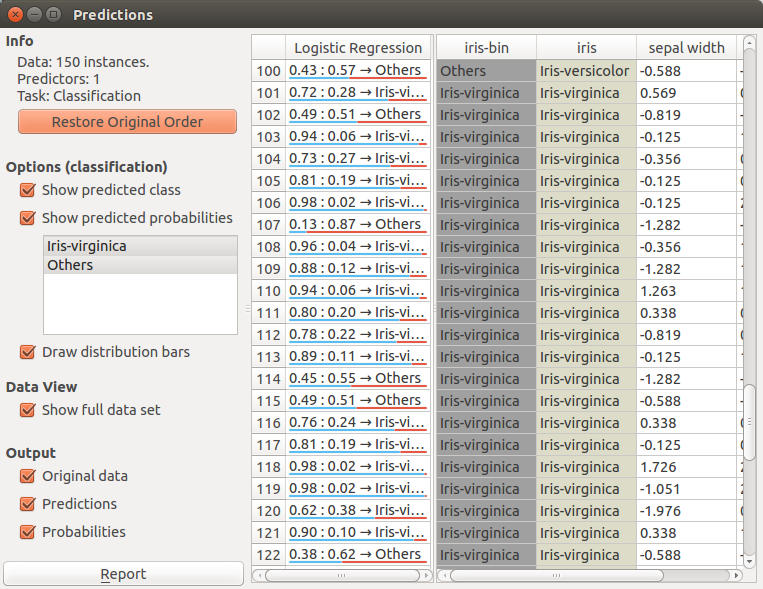If we want to demonstrate linear regression we can change data set to Housing. That data set has a continuous class variable. When using linear regression we can select only one feature what means that our function is linear. The another parameter that is plotted in the graph is intercept of a linear function.

This time we selected INDUS as a independent variable. In widget we can make same actions as before. In the end we can also check predictions for each point with Predictions widget. And coefficients of linear regression in a Data Table.